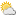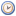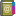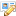出发城市： A 阿克苏 A 阿勒泰 A 安康 A 安庆 A 鞍山 B 百色 B 保山 B 包头 B 北安 B 北海 B 北京 B 北京南苑 B 蚌埠 C 长春 C 常德 C 长沙 C 常熟 C 长治 C 常州 C 朝阳 C 成都 C 赤峰 C 重庆 D 大理 D 大连 D 丹东 D 大同 D 达县 D 东营 D 敦煌 E 恩施 F 阜阳 F 福州 G 赣州 G 格尔木 G 广汉 G 广州 G 桂林 G 贵阳 H 海口 H 海拉尔 H 海宁 H 哈密 H 邯郸 H 杭州 H 汉中 H 哈尔滨 H 合肥 H 黑河 H 衡阳 H 呼和浩特 H 和田 H 长沙/黄花 H 黄山 H 黄岩 H 呼伦贝尔 J 佳木斯 J 吉安 J 嘉峪关 J 吉林 J 济南 J 景德镇 J 井冈山 J 济宁 J 晋江 J 锦州 J 九江 J 酒泉 J 九寨沟 K 喀什 K 克拉玛依 K 库尔勒 K 昆明 L 兰州 L 连云港 L 连州 L 丽江 L 临沧 L 临沂 L 丽水 L 柳州 M 澳门 M 满洲里 M 绵阳 M 牡丹江 N 南昌 N 南充 N 南京 N 南宁 N 南通 N 南阳 N 宁波 P 攀枝花 P 普宁 Q 青岛 Q 秦皇岛 Q 庆阳 Q 齐齐哈尔 S 三亚 S 上海虹桥 S 上海浦东 S 汕头 S 沈阳 S 深圳 S 石家庄 S 十堰 S 思茅 S 绥芬河 T 塔城 T 太原 T 塔什干 T 天津 T 铜仁 U 乌兰浩特 U 乌鲁木齐 W 潍坊 W 威海 W 文山州 W 温州 W 乌海 W 武汉 W 芜湖 W 吴江 W 武进 W 五台山 W 武威 W 无锡 W 武穴 W 武夷山 W 五指山 W 梧州 X 厦门 X 西安 X 西昌 X 锡林浩特 X 西宁 X 新密 X 新乡 X 信阳 X 信宜 X 新余 X 忻州 X 西双版纳 X 宣城 X 许昌 X 徐州 Y 雅安 Y 延安 Y 盐城 Y 雁荡山 Y 荥阳 Y 濮阳 Y 溧阳 Y 阳春 Y 阳江 Y 阳泉 Y 阳朔 Y 扬中 Y 扬州 Y 延吉 Y 烟台 Y 宜宾 Y 宜昌 Y 宜春 Y 伊春 Y 银川 Y 营口 Y 鹰潭 Y 伊宁 Y 义乌 Y 宜兴 Y 益阳 Y 仪征 Y 宜州 Y 永安 Y 永康 Y 永州 Y 元谋 Y 岳阳 Y 榆林 Y 玉林 Y 玉门 Y 运城 Y 运城 Y 云浮 Y 玉树州 Y 玉溪 Y 禹州 Z 枣阳 Z 枣庄 Z 扎兰屯 Z 张家港 Z 张家界 Z 张家口 Z 漳平 Z 张掖 Z 漳州 Z 湛江 Z 肇庆 Z 昭通 Z 郑州 Z 镇江 Z 阆中 Z 中山 Z 钟祥 Z 嵊州 Z 涿州 Z 儋州 Z 亳州 Z 兖州 Z 邳州 Z 周口 Z 舟山 Z 周庄 Z 诸暨 Z 珠海 Z 驻马店 Z 株洲 Z 株州 Z 淄博 Z 自贡 Z 资兴 Z 资阳 Z 晋江 Z 邹城 Z 遵化 Z 遵义 到达城市： A 阿克苏 A 阿勒泰 A 安康 A 安庆 A 鞍山 B 百色 B 保山 B 包头 B 北安 B 北海 B 北京 B 北京南苑 B 蚌埠 C 长春 C 常德 C 长沙 C 常熟 C 长治 C 常州 C 朝阳 C 成都 C 赤峰 C 重庆 D 大理 D 大连 D 丹东 D 大同 D 达县 D 东营 D 敦煌 E 恩施 F 阜阳 F 福州 G 赣州 G 格尔木 G 广汉 G 广州 G 桂林 G 贵阳 H 海口 H 海拉尔 H 海宁 H 哈密 H 邯郸 H 杭州 H 汉中 H 哈尔滨 H 合肥 H 黑河 H 衡阳 H 呼和浩特 H 和田 H 长沙/黄花 H 黄山 H 黄岩 H 呼伦贝尔 J 佳木斯 J 吉安 J 嘉峪关 J 吉林 J 济南 J 景德镇 J 井冈山 J 济宁 J 晋江 J 锦州 J 九江 J 酒泉 J 九寨沟 K 喀什 K 克拉玛依 K 库尔勒 K 昆明 L 兰州 L 连云港 L 连州 L 丽江 L 临沧 L 临沂 L 丽水 L 柳州 M 澳门 M 满洲里 M 绵阳 M 牡丹江 N 南昌 N 南充 N 南京 N 南宁 N 南通 N 南阳 N 宁波 P 攀枝花 P 普宁 Q 青岛 Q 秦皇岛 Q 庆阳 Q 齐齐哈尔 S 三亚 S 上海虹桥 S 上海浦东 S 汕头 S 沈阳 S 深圳 S 石家庄 S 十堰 S 思茅 S 绥芬河 T 塔城 T 太原 T 塔什干 T 天津 T 铜仁 U 乌兰浩特 U 乌鲁木齐 W 潍坊 W 威海 W 文山州 W 温州 W 乌海 W 武汉 W 芜湖 W 吴江 W 武进 W 五台山 W 武威 W 无锡 W 武穴 W 武夷山 W 五指山 W 梧州 X 厦门 X 西安 X 西昌 X 锡林浩特 X 西宁 X 新密 X 新乡 X 信阳 X 信宜 X 新余 X 忻州 X 西双版纳 X 宣城 X 许昌 X 徐州 Y 雅安 Y 延安 Y 盐城 Y 雁荡山 Y 荥阳 Y 濮阳 Y 溧阳 Y 阳春 Y 阳江 Y 阳泉 Y 阳朔 Y 扬中 Y 扬州 Y 延吉 Y 烟台 Y 宜宾 Y 宜昌 Y 宜春 Y 伊春 Y 银川 Y 营口 Y 鹰潭 Y 伊宁 Y 义乌 Y 宜兴 Y 益阳 Y 仪征 Y 宜州 Y 永安 Y 永康 Y 永州 Y 元谋 Y 岳阳 Y 榆林 Y 玉林 Y 玉门 Y 运城 Y 运城 Y 云浮 Y 玉树州 Y 玉溪 Y 禹州 Z 枣阳 Z 枣庄 Z 扎兰屯 Z 张家港 Z 张家界 Z 张家口 Z 漳平 Z 张掖 Z 漳州 Z 湛江 Z 肇庆 Z 昭通 Z 郑州 Z 镇江 Z 阆中 Z 中山 Z 钟祥 Z 嵊州 Z 涿州 Z 儋州 Z 亳州 Z 兖州 Z 邳州 Z 周口 Z 舟山 Z 周庄 Z 诸暨 Z 珠海 Z 驻马店 Z 株洲 Z 株州 Z 淄博 Z 自贡 Z 资兴 Z 资阳 Z 晋江 Z 邹城 Z 遵化 Z 遵义 出发日期： 航空公司： 不限航空公司 西奈航空公司 国际航空公司 北方航空公司 南方航空公司 鹰联航空公司 上海航空公司 厦门航空公司 东方航空公司 山东航空公司 南方航空运输 联合航空公司 深圳航空公司 穆克航空公司 四川航空公司 上海吉祥航空 奥凯航空公司 中国联合航空 东星航空公司 祥鹏航空公司 华夏航空公司 大新华快运 重庆航空公司 西部航空公司天气预报航空预定列车时刻长途汽车出境宝典签证办理
• 特价航空
--

## 推荐酒店Tel: 028-69696333

Tel: 028-69696444